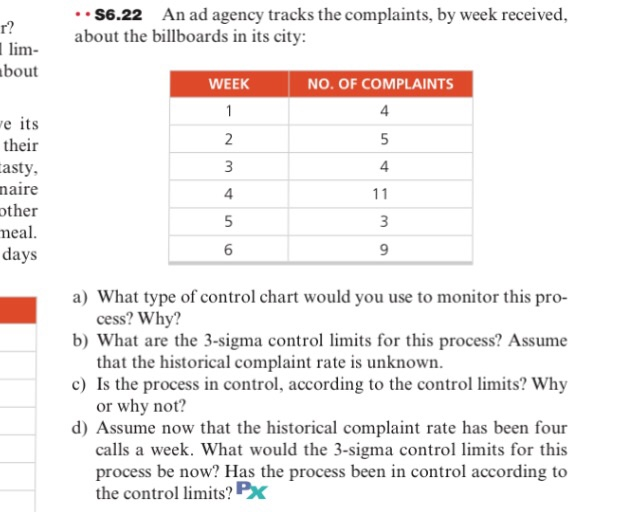# High quality homework help from top writers

Every paper is written from scratch. Our top homework writers will please you with excellent quality and timing for your paperAn ad agency tracks the complaints, by week received, about the billboards in its city: a) What type of control chart would you use to monitor this process? Why? b) What are the 3-sigma control limits for this process? Assume that the historical complaint rate is unknown. c) Is the process in control, according to the control limits? Why or why not? d) Assume now that the historical complaint rate has been four calls a week. What would the 3-sigma control limits for this process be now? Has the process been in control according to the control limits?

Don't use plagiarized sources. Get Your Custom Essay on
From \$8/Page

Choice of C hart will be the appropriate control chart to monitor this process.

C chart is used when counting total count of defects ( here that is ‘complaints’) per unit ( here that is ‘week)and is appropriate when the sample size is uniform . Here sample size for each case is “Week” and hence has an uniform sample size.

In case sample size was not uniform , c chart could not have been used and instead u chart would have been used.

Let , Cbar = Average number of complaints per week

Therefore, Cbar = ( 4 + 5 + 4 + 11 + 3 + 9) / 6 = 6

Thus 3 sigma control limits are :

Upper Control Limit ( UCL )

= Cbar + 3 x square root ( Cbar)

= 6 + 3x square root ( 6)

= 6 + 3 x 2.45

UPP

= 13.35

Centerline ( CL) =Cbar = 6

Lower control Limit ( LCL)

= Cbar – 3 x square root ( Cbar)

= 6 – 3 x 2.45

= 6 – 7.35

= – 1.35

However since derived result for CL is negative number, we must consider LCL = 0

 3 SIGMA UPPER CONTROL LIMIT ( UCL ) = 13.35 CENTERLINE = 6 3 SIGMA LOWER CONTROL LIMIT ( LCL) = 0

The data on number of complaints are : 4. 5, 4 , 11, 3 and 9 . It can be seen that all these data are within UCL of 13.35 and LCL of 0 . Therefore, it can be concluded that the process is in control.

Now the historical complaint rate = 4 calls a week

Therefore ,

C bar = Average of all historical complaint rates each of which is 4 = 4

Therefore,

3 Sigma Upper Control Limit ( UCL )

= Cbar + 3x Square root ( Cbar)

= 4 + 3 x Square root( 4)

= 4 + 6

= 10

Centerline = Cbar = 4

3 sigma Lower control Limit

= Cbar – 3 x Square root ( Cbar )

= 4 – 3 x 2

= 4 – 6

= – 2

However since LCL cannot be a negative number, we shall consider LCL = 0

Thus :

 Upper Control Limit = 10 Center Line = 4 Lower Control Limit = 0

It is to be noted that there is complaint data e.g 11 which is > UCL . Therefore it can be concluded that the process is out of control.

selected t-accounts of moore company are given below for the just completed year: